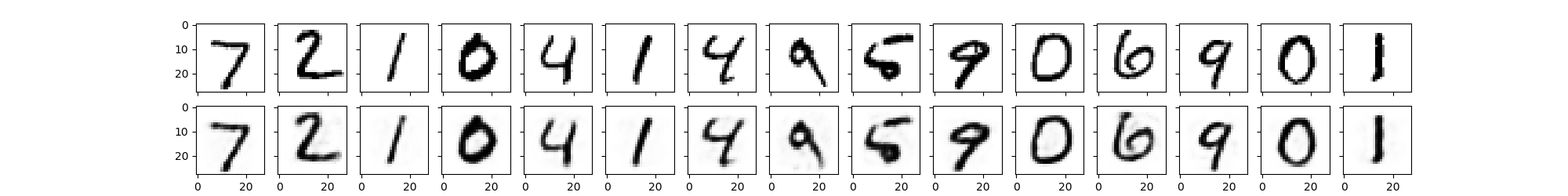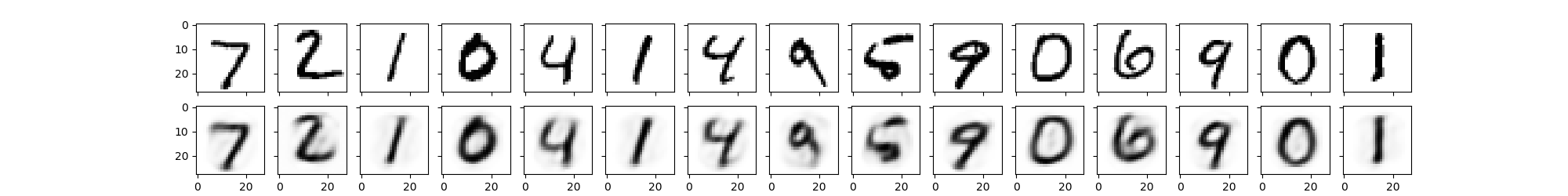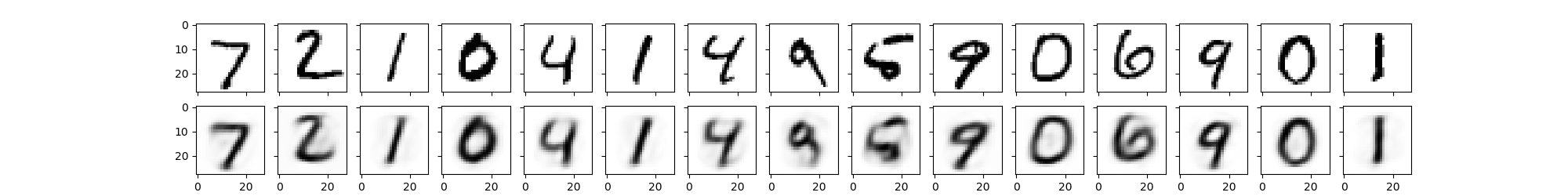# 自编码器的原理及实现

## 1. 什么是自编码器

1. 先对无标注的数据进行自编码器的训练，然后将有标注的数据第一步输入自编码器，自编码器的输出输入到神经网络之中，达到提升网络输出精度的目的。

2. 用于神经网络权重的初始化。

$x\overset{f(x)}{\rightarrow}h\overset{g(x)}{\rightarrow}x'$

$x\approx x'$

$x'=g(f(x))$

## 损失函数的设计

$L(x,g(f(x))=E_{x\sim~p_{data}}\left\|x-g(f(x))\right\|^2$

L1正则化仅仅作用于编码，因为我们关心的是编码的过程，解码器只是方便显示编码之后的结果。
$\Omega(h)=\lambda\sum\nolimits_{i}\left|h_i\right|$
$h_i$是第$i$个神经元的激励值。

$\hat{\rho}_j=\frac{1}{N}\sum\nolimits_{i=1}^{N}{h}^{(i)}_j$
$i$求和表示对训练集（N个样本）的所有输入取均值，j代表第几个隐层神经元，激励值通常在$0\sim1$之间。对稀疏性的约束就是令神经元的平均活跃度接近稀疏性系数$\rho:\hat{\rho}_j\approx\rho$这个系数通常取接近0的值，从而约束隐层神经元的活跃程度，可以把$\rho$理解成某个神经元被激活的概率。

$\sum\nolimits_{j=1}^{M}KL(\rho\parallel\hat{\rho}_j)=\sum\nolimits_{j=1}^{M}[\rho\cdot\log\frac{p}{\hat{\rho}_j}+(1-\rho)\cdot\log\frac{1-p}{1-\hat{\rho}_j}]$
KLD是一种衡量两个分布之间差异的方法，式中的$\rho$$\hat{\rho}_j$分别表示期望和实际的隐层神经元的输出两点分布（两点分别代表饱和和睡眠）的均值和期望。

L1：
$L(x,g(f(x))=E_{x\sim~p_{data}}\left\|x-g(f(x))\right\|^2+\lambda\sum\nolimits_{i}\left|h_i\right|$
KLD：
$L(x,g(f(x))=E_{x\sim~p_{data}}\left\|x-g(f(x))\right\|^2+\beta\sum_{j=1}^{M}[\rho\cdot\log\frac{p}{\hat{\rho}_j}+(1-\rho)\cdot\log\frac{1-p}{1-\hat{\rho}_j}]$

Sigmoid Sigmoid True False True
Relu Softplus True True False

# coding:utf-8

import tensorflow as tf
import tensorlayer as tl
from tensorlayer.layers import *
import numpy as np
import matplotlib.pylab as plt

learning_rate = 0.0001
lambda_l2_w = 0.01
n_epochs = 100
batch_size = 128
print_interval = 200

hidden_size = 196
input_size = 784
image_width = 28
model = 'sigmoid'

X_train, y_train, X_val, y_val, X_test, y_test = tl.files.load_mnist_dataset(path='./data/')

x = tf.placeholder(tf.float32,shape=[None,784],name='x')

print('Build Network')
if model=='relu':
net = InputLayer(x, name='input')
net = DenseLayer(net,n_units=hidden_size,act=tf.nn.relu, name='relu1')
encode_img = net.outputs
recon_layer1 = DenseLayer(net,n_units=input_size,act=tf.nn.softplus,name='recon_layer1')

if model=='sigmoid':
net = InputLayer(x, name='input')
net = DenseLayer(net,n_units=hidden_size,act=tf.nn.sigmoid, name='sigmoid1')
encode_img = net.outputs
recon_layer1 = DenseLayer(net,n_units=input_size,act=tf.nn.sigmoid,name='recon_layer1')

y = recon_layer1.outputs
train_params = recon_layer1.all_params[-4:]

mse = tf.reduce_sum(tf.squared_difference(y,x),1)
mse = tf.reduce_mean(mse)

# w1 和 w2 采用L2正则化
L2_w = tf.contrib.layers.l2_regularizer(lambda_l2_w)(train_params)+\
tf.contrib.layers.l2_regularizer(lambda_l2_w)(train_params)

# 稀疏性约束
activation_out = recon_layer1.all_layers[-2]
L1_a = 0.001 * tf.reduce_mean(activation_out)

# 相对熵（KLD）
beta = 0.5
rho = 0.15
p_hat = tf.reduce_mean(activation_out,0)
KLD = beta * tf.reduce_sum(rho * tf.log(tf.divide(rho,p_hat)) +
(1-rho) * tf.log((1-rho)/(tf.subtract(float(1),p_hat))))

# 联合损失函数
if model=='sigmoid':
cost = mse + L2_w + KLD
if model=='relu':
cost = mse + L2_w + L1_a

# 定义优化器
saver = tf.train.Saver()

# 模型训练
total_batch = X_train.shape // batch_size

with tf.Session() as sess:
sess.run(tf.global_variables_initializer())

for epoch in range(n_epochs):
avg_cost = 0
for i in range(total_batch):
batch_x,batch_y =X_train[i*batch_size:(i+1)*batch_size],y_train[i*batch_size:(i+1)*batch_size]
batch_x = np.array(batch_x).astype(np.float32)
batch_cost, _ = sess.run([cost, train_op], feed_dict={x: batch_x})
if not i % print_interval:
print('Minibatch: %03d | Cost:  %.3f' % (i + 1, batch_cost))
print('Epoch:   %03d | AvgCost:  %.3f' % (epoch + 1, avg_cost / i + 1))
saver.save(sess,save_path='./model/3-101.ckpt')

# 恢复参数
n_images=15
fig,axes=plt.subplots(nrows=2,ncols=n_images,sharex=True,sharey=True,figsize=(20,2.5))

test_images = X_test[:n_images]

with tf.Session() as sess:
# 加载训练好的模型
saver.restore(sess,save_path='./model/3-101.ckpt')

# 获取重构参数
decoded = sess.run(recon_layer1.outputs,feed_dict={x:test_images})
# 恢复编码器的权重参数
if model=='relu':
weights = sess.run(tl.layers.get_variables_with_name('relu1/W:0',False,True))
if model=='sigmoid':
weights = sess.run(tl.layers.get_variables_with_name('sigmoid1/W:0',False,True))

# 获取解码器的权重参数
recon_weights = sess.run(tl.layers.get_variables_with_name('recon_layer1/W:0',False,True))
recon_bias = sess.run(tl.layers.get_variables_with_name('recon_layer1/b:0',False,True))
for i in range(n_images):
for ax,img in zip(axes,[test_images,decoded]):
ax[i].imshow(img[i].reshape(image_width,image_width),cmap='binary')
plt.show()


MSEMSE+L2MSE+L2+KLD